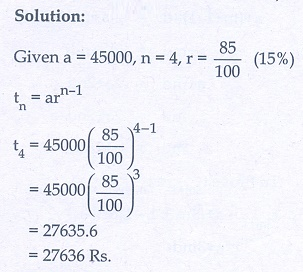Home | | Maths 10th Std | Exercise: Numbers and Sequences

# Exercise: Numbers and Sequences

Maths Book back answers and solution for Exercise questions - Mathematics : Numbers and Sequences: Exercise Problem Questions with Answer

Unit Exercise

1. Prove that n2 - n divisible by 2 for every positive integer n.2. A milk man has 175 litres of cow’s milk and 105 litres of buffalow’s milk. He wishes to sell the milk by filling the two types of milk in cans of equal capacity. Calculate the following (i) Capacity of a can (ii) Number of cans of cow’s milk (iii) Number of cans of buffalow’s milk.3. When the positive integers ab and c are divided by 13 the respective remainders are 9, 7 and 10. Find the remainder when a + 2b + 3c is divided by 13.4. Show that 107 is of the form 4q + 3 for any integer q.5. If (m + 1)th term of an A.P. is twice the (n + 1)th term, then prove that (3m+1)th term is twice the (m + n + 1)th term.6. Find the 12th term from the last term of the A. P - 2, -4,- 6, ... -100 .7. Two A.P.’s have the same common difference. The first term of one A.P. is 2 and that of the other is 7. Show that the difference between their 10thterms is the same as the difference between their 21st terms, which is the same as the difference between any two corresponding terms.8. A man saved ₹16500 in ten years. In each year after the first he saved ₹100 more than he did in the preceding year. How much did he save in the first year?9. Find the G.P. in which the 2nd term is √6 and the 6th term is 9√6 .10. The value of a motor cycle depreciates at the rate of 15% per year. What will be the value of the motor cycle 3 year hence, which is now purchased for ₹ 45,000?2. (i) 35 litres (ii) 5 (iii) 3

3. 1

6. -78

8. ₹1200

9. √2, √6 , 3√2 , …

10. ₹27636

Tags : Problem Questions with Answer, Solution | Mathematics , 10th Mathematics : UNIT 2 : Numbers and Sequences
Study Material, Lecturing Notes, Assignment, Reference, Wiki description explanation, brief detail
10th Mathematics : UNIT 2 : Numbers and Sequences : Exercise: Numbers and Sequences | Problem Questions with Answer, Solution | Mathematics